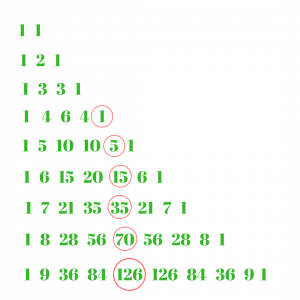# Pentatope number

Given a number n, find the nth Pentatope number. A pentatope number is represented by the fifth number in any row of Pascal’s Triangle. As it is fifth number, it should start from row having at least 5 numbers. So, it starts from row 1 4 6 4 1. The formula for the nth pentatopic number is: n (n+1) (n+2) (n+3) / 24

Starting Pentatope numbers are : 1, 5, 15, 35, 70, 126, 210, 330, 495…..

Pentatope Numbers:In the above figure, red color circled numbers are pentatope numbers.

Examples:

```Input : 4
Output : 35

Input : 8
Output : 330
```

Below is the implementation for nth Pentatope Number :

## C++

 `// CPP Program to find the ` `// nth Pentatope number ` `#include ` `using` `namespace` `std; ` ` `  `// function for Pentatope ` `// number ` `int` `Pentatope_number(``int` `n) ` `{ ` `    ``// formula for find Pentatope ` `    ``// nth term ` `    ``return` `n * (n + 1) * (n + 2) * (n + 3) / 24; ` `} ` ` `  `// Driver Code ` `int` `main() ` `{ ` `    ``int` `n = 7; ` `    ``cout << n << ``"th Pentatope number :"`  `         ``<< Pentatope_number(n) << endl; ` ` `  `    ``n = 12; ` `    ``cout << n << ``"th Pentatope number :"`  `         ``<< Pentatope_number(n) << endl; ` ` `  `    ``return` `0; ` `} `

## Java

 `// Java Program to find the nth Pentatope ` `// number ` `import` `java.io.*; ` ` `  `class` `GFG { ` ` `  `    ``// function for Pentatope ` `    ``// number ` `    ``static` `int` `Pentatope_number(``int` `n) ` `    ``{ ` `         `  `        ``// formula for find Pentatope ` `        ``// nth term ` `        ``return` `n * (n + ``1``) * (n + ``2``) *  ` `                         ``(n + ``3``) / ``24``; ` `    ``} ` `     `  `    ``// Driver Code ` `    ``public` `static` `void` `main (String[] args) ` `    ``{ ` `        ``int` `n = ``7``; ` `        ``System.out.println( n + ``"th "` `                   ``+ ``"Pentatope number :"` `                  ``+ Pentatope_number(n)); ` `                   `  `        ``n = ``12``; ` `        ``System.out.println( n + ``"th "` `                  ``+ ``"Pentatope number :"` `                 ``+ Pentatope_number(n)); ` `    ``} ` `} ` ` `  `// This code is contributed by anuj_67. `

## Python3

 `# Python3 program to find ` `# nth Pentatope number ` ` `  `# Function to calculate ` `# Pentatope number ` `def` `Pentatope_number(n): ` ` `  `    ``# Formula to calculate nth ` `    ``# Pentatope number ` `    ``return` `(n ``*` `(n ``+` `1``) ``*` `(n ``+` `2``)  ` `                 ``*` `(n ``+` `3``) ``/``/` `24``) ` ` `  `# Driver Code ` `n ``=` `7` `print``(``"%sth Pentatope number : "` `%``n, ` `                    ``Pentatope_number(n)) ` `n ``=` `12` `print``(``"%sth Pentatope number : "` `%``n, ` `                    ``Pentatope_number(n)) ` ` `  `# This code is contributed by ajit.      `

## C#

 `// C# Program to find the nth Pentatope  ` `// number ` `using` `System; ` ` `  `class` `GFG { ` ` `  `    ``// function for Pentatope ` `    ``// number ` `    ``static` `int` `Pentatope_number(``int` `n) ` `    ``{ ` `         `  `        ``// formula for find Pentatope ` `        ``// nth term ` `        ``return` `n * (n + 1) * (n + 2) *  ` `                         ``(n + 3) / 24; ` `    ``} ` `     `  `    ``// Driver Code ` `    ``public` `static` `void` `Main () ` `    ``{ ` `        ``int` `n = 7; ` `        ``Console.WriteLine( n + ``"th "` `                ``+ ``"Pentatope number :"` `               ``+ Pentatope_number(n)); ` `                `  `        ``n = 12; ` `        ``Console.WriteLine( n + ``"th "` `                ``+ ``"Pentatope number :"` `               ``+ Pentatope_number(n)); ` `    ``} ` `} ` ` `  `// This code is contributed by anuj_67. `

## PHP

 ` `

Output :

```7th Pentatope number : 210
12th Pentatope number : 1365
```

References : https://en.wikipedia.org/wiki/Pentatope_number/

My Personal Notes arrow_drop_upIf you like GeeksforGeeks and would like to contribute, you can also write an article using contribute.geeksforgeeks.org or mail your article to contribute@geeksforgeeks.org. See your article appearing on the GeeksforGeeks main page and help other Geeks.

Please Improve this article if you find anything incorrect by clicking on the "Improve Article" button below.

Improved By : vt_m, jit_t

Article Tags :
Practice Tags :

Be the First to upvote.

Please write to us at contribute@geeksforgeeks.org to report any issue with the above content.